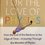# Use of complex numbers in calculus.

Hi, you might have come across:$\displaystyle \int e^{ax}\cos bx$ dx,

How do you solve it? You might use integration by parts, and also complex numbers, and I find use of complex numbers interesting!

Say $A = \displaystyle \int e^{ax}\cos bx$dx,

and $B = \displaystyle \int e^{ax}\sin bx$ dx

Hence, $A + iB = \displaystyle \int e^{ax} (\cos bx + i \sin bx)$dx = $\displaystyle \int e^{ax} (e^{i bx})$dx

= $\displaystyle \int e^{(a+ib)x}$dx

= $\displaystyle \frac{e^{(a+ib)x}}{a+ib}$

= $\displaystyle \frac{e^{(a+ib)x}(a - ib)}{a^2 + b^2}$

$\Rightarrow A + iB = z = \displaystyle \frac{e^{ax} (\cos bx + i \sin bx)(a - ib)}{a^2+b^2}$

Clearly,

$A = \text{Re}(z) = \displaystyle \frac{e^{ax}}{a^2+b^2} (a \cos bx + b \sin bx)$

$B = \text{Im}(z) = \displaystyle \frac{e^{ax}}{a^2+b^2} (a \sin bx - b \cos bx)$

You can try to find this definite integral:

Problem: $\displaystyle \int_{0}^{\pi} e^{(\cos x)} \cos(\sin x)$ dx7 years, 7 months ago

This discussion board is a place to discuss our Daily Challenges and the math and science related to those challenges. Explanations are more than just a solution — they should explain the steps and thinking strategies that you used to obtain the solution. Comments should further the discussion of math and science.

When posting on Brilliant:

• Use the emojis to react to an explanation, whether you're congratulating a job well done , or just really confused .
• Ask specific questions about the challenge or the steps in somebody's explanation. Well-posed questions can add a lot to the discussion, but posting "I don't understand!" doesn't help anyone.
• Try to contribute something new to the discussion, whether it is an extension, generalization or other idea related to the challenge.

MarkdownAppears as
*italics* or _italics_ italics
**bold** or __bold__ bold
- bulleted- list
• bulleted
• list
1. numbered2. list
1. numbered
2. list
Note: you must add a full line of space before and after lists for them to show up correctly
paragraph 1paragraph 2

paragraph 1

paragraph 2

[example link](https://brilliant.org)example link
> This is a quote
This is a quote
    # I indented these lines
# 4 spaces, and now they show
# up as a code block.

print "hello world"
# I indented these lines
# 4 spaces, and now they show
# up as a code block.

print "hello world"
MathAppears as
Remember to wrap math in $$ ... $$ or $ ... $ to ensure proper formatting.
2 \times 3 $2 \times 3$
2^{34} $2^{34}$
a_{i-1} $a_{i-1}$
\frac{2}{3} $\frac{2}{3}$
\sqrt{2} $\sqrt{2}$
\sum_{i=1}^3 $\sum_{i=1}^3$
\sin \theta $\sin \theta$
\boxed{123} $\boxed{123}$

Sort by:

Short and Sweet! :)

- 7 years, 7 months ago

Thanks!

- 7 years, 7 months ago

$cos { (\sin { x) } } =\quad ({ e }^{ isinx }+{ e }^{ -isinx })/2\\ { e }^{ cosx }\cos { (\sin { x) } } =\quad ({ e }^{ cosx+isinx }+{ e }^{ cosx-isinx })/2=({ e }^{ { e }^{ ix } }+{ e }^{ { e }^{ -ix } })/2\\ =((1+\frac { { e }^{ ix } }{ 1! } +\frac { { e }^{ i2x } }{ 2! } +...)+(1+\frac { { e }^{ -ix } }{ 1! } +\frac { { e }^{ -2ix } }{ 2! } +...))/2$

In 2 more steps you will get the answer. The answer in this case is $pi$

- 7 years, 2 months ago

Solve his other question too: Problem without words !

- 7 years, 7 months ago

Hi Jatin! Thanks for the great post, but just one thing I was wondering that incorporating i, the complex number, and using the usual laws of Calculus, is it mathematically correct, I mean the laws of calculus is for reals. I may be fundamentally wrong somewhere but I need the answer.

- 7 years, 6 months ago

Awesome article.

By using your method I arrive at a step from where I can't proceed further.

The step is: $A+iB=\int (e)^{e^{ix}}\,dx$, where $A=\int (e)^{(cos x)}cos(sin x)\,dx$ and $B=\int (e)^{(cos x)}sin(sin x)\,dx$.

How to do after this?Precisely,what's the real part?

- 7 years, 7 months ago

Hi, Bhargav, you are close, try to use expansion for $e^x$.

- 7 years, 7 months ago

Is the answer $\pi$?

- 7 years, 7 months ago

I reach the same answer but is it possible to evaluate $B$?

- 7 years, 7 months ago

You came our with a simple and an interesting solution the technique that triggered me was to solve it using By Parts Method but have to admit your approach was far far better than mines Can you tell me how do you get such ideas at and tender age of 15(Just Asking)?

- 7 years, 7 months ago

Hi, solving this integral using complex numbers is well(not very much well though) known. I did not come up with it myself.

- 7 years, 7 months ago

Yeah, actually the same technique's been discussed with us at our insti as well... anyways, it's really good...

- 7 years, 7 months ago

Awesome!!

- 7 years, 7 months ago

-e^{-1} -1 is the ans

- 7 years, 6 months ago

great..:-)

- 7 years, 5 months ago

Too beautiful

- 7 years, 2 months ago

- 7 years, 2 months ago

Thanks a ton..!

- 7 years, 2 months ago

Easy approach....nice trick...

- 7 years, 2 months ago

Interesting method!

- 7 years, 1 month ago

Finally I got it! :)

- 6 years, 8 months ago

THANKS. VERY POWERFULL METHOD-CAN BE TACKLED ANY COMPLICATED INTEGRALS LIKE THIS ONE.

- 6 years, 8 months ago

It is an amazing approach to solve such problems.

- 6 years, 7 months ago

$W0w$ $£xcellent approach$

- 6 years, 4 months ago